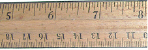Name:    2nd Grade Measurement and Data- Measure and estimate lengths in standard units, Standard 1, Pre Test 1 Measure the length of an object by selecting and using appropriate tools such as rulers, yardsticks, meter sticks, and measuring tapes.

Multiple Choice
Identify the choice that best completes the statement or answers the question.

1.

Which measuring tool would you use to measure this item?a. measuring cup c. scale b. ruler d. none of the above

2.

Which measuring tool would you use to measure the green field inside the stadium?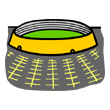a. ruler c. measuring tape b. scale d. none of the above

3.

Which measuring tool would you use to measure the length of the bowling alley lanea. ruler c. scale b. yard stick d. none of the above

4.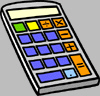What measuring tool would be the best one for measuring the calculator?
 a. scale c. ruler b. yard stick d. measuring tape

5.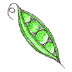What measuring tool would be the best one for measuring ONE pea in the pod?
 a. yard stick c. ruler b. measuring tape d. metric ruler

6.How long is one side of this box?
 a. 1/2 inch c. 1 1/2 inches b. 4 inches d. 2 inches

7.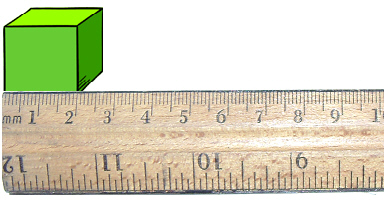How long is one side of this box?
 a. 1/2 inch c. 3 centimeters b. 2 centimeters d. 2 inches

8.

Which tool would you use to measure this door?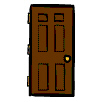a.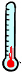c.b.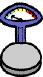d.